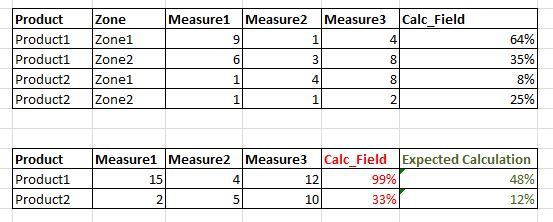## How can I calucalate ratio of two measures?

Hi,

I'm using SAS VA 7.3.

I have to show ratio of fields in a table.

For this I have created a new calculated measure with following formula:

Calc_Field = Measure1 / (Measure1 + Measure2 + Measure3)

It works fine if I add all categories on the report table.

But if I remove any category, VA sums the values of Calc_Field instead of recalculate the formula.I think in backend SAS groups by all category variables and based on this it sums up all measure variables.

So basically it is doing sum(Measure1), sum(Measure2), sum(Measure3), sum(Calc_Field).

But I want value of Calc_Field to be calculated based on defined formula once other fields are grouped.

I tried both List table and CrossTab. I also tried different aggregation property of this calculated field but no luck.

Any help or suggestion will be much appreciated.

Thanks

1 ACCEPTED SOLUTION

Accepted Solutions

Hi there,

Thanks,

Teja Surapaneni

3 REPLIES 3

Hi there,

Thanks,

Teja Surapaneni

## Re: How can I calucalate ratio of two measures?

Thanks a lot @TejaSurapaneni it worked and I'm getting the expected result.

Just one minor problem.

If one of my measure is missing then aggregated formula is also returning a missing value.

In base SAS we can use Sum(var1,var2) function to avoid this problem.

But in VA it is giving me syntax error.

What should I modify in this formula to add numbers with missing value?

``Sum [_ByGroup_] ('M1'n) / ( Sum [_ByGroup_] ('M2'n) + Sum [_ByGroup_] ('M3'n) )``

Any suggestion?

## Re: How can I calucalate ratio of two measures?

For a quick fix I replaced all missing values to 0 (zeros).

It worked for now.

But I'll be looking for a good solution of this problem.

I'm sure there should be some way to negate missing value effect.

Discussion stats
• 3 replies
• 1502 views
• 1 like
• 2 in conversation